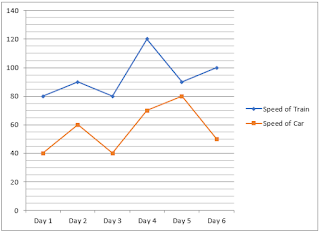SBI PO Quantitative Aptitude Quiz: 22nd May

SBI PO Quantitative Aptitude Quiz

Here, we are providing free crash course for SBI PO as there is left not enough time to deal in details. The questions asked in the quantitative aptitude section are calculative and very time-consuming. But once dealt with proper strategy, speed, and accuracy, this section can get you the maximum marks in the examination. Following is the Quantitative Aptitude quiz to help you practice with the best of latest pattern questions

Directions (1-5): What approximate value should come in place of the question mark (?) in the following questions?

Q1. 149.98% of 30.03 + 25.05% of 139.96 = ?

85
70
65
80
75
0.12
1.2
2.4
12
24

Q3. 1444.9998 × 80.0001 ÷ 99.999 = (?)²

34
30
24
12
17
14
15
18
17
16
17
20
14
24
10

Directions (6-10): Refer the following line graph and answer the questions based on it.

The line graph shows the speeds (in km/h) of car and train during on six days in a week.Q6. What is the average speed of the train on Day 1, Day 3 and Day 4 together if the train travelled same distance on each of these days?

95 km/h
90 km/h
85 km/h
100 km/h
None of these

Q7. On day 5, the train covered a distance of 300 km while the car covered 240 km. What is the ratio of time taken by the car to the time taken by the train on that day?

6 : 7
7 : 6
10 : 9
9 : 10
None of these

Q8. If the time taken by the car is thrice to the time taken by the train on day 3, then the distance covered by the car is how much percent more than the distance covered by the train on that day?

50%
40%
60%
30%
None of these

Q9. For which day, the percentage increase/decrease in the speed of train from the previous day is the maximum?

Day 4 and 5
Day 5
Day 4
Day 2 and 4
None of these

Q10. If both the train and the car travelled for 3 hours 20 minutes each on day 2, then what is the difference of the distances travelled by the train and the car on that day?

60 km
120 km
90 km
100 km
Cannot be determined

Q11. Priya can do ½ of the work in 10 days while Pooja can do ⅓ of the work in 10 days. How long it will take for both of them to finish the work if Priya started the work and they work on alternative days.

15 days
18 days
21 days
24 days
27 days

Q12. In a football championship, there were 210 matches played. Every two teams played one match with each other. The number of teams participating in the championship is

20
21
19
18
17

Q13. Ram bought a bike at 20% discount on MRP. After 1 year Ram sell the bike to Ramesh at 10% loss. After 1 year more Ramesh sell the bike at 20% profit to Ranjan. If Ranjan paid Rs. 1,29,600, then find the M.R.P. of the bike ?

1,50,000
2,25,000
1,40,000
2,00,000
1,80,000

Q14. If on a certain amount of sum, simple interest is 600 at 8% rate annually for 3 years then what will be the CI on that sum for 3 years with 8% rate annually?

646.32
640.20
654.35
649.28
None of these

Q15. The average weight of 25 students is 34 kg. It was found later that the weight of a student is misread as 86 kg instead of 36 kg, then find out the correct average ?

30
31
32
33
35

WATCH VIDEO SOLUTIONS HERE